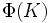# Finite-Frattini-realizable group

## Contents

BEWARE! This term is nonstandard and is being used locally within the wiki. [SHOW MORE]
This article defines a property that can be evaluated for finite groups (and hence, a particular kind of group property)
View other properties of finite groups OR View all group properties
This term is related to the problem of realization related to the following subgroup-defining function: Frattini subgroup
Realization problems are usually about which groups can be realized as subgroups/quotients related to a subgroup-defining function.
View other terminology related to realization problems for Frattini subgroup OR View facts related to them

## Definition

### Symbol-free definition

A finite group is termed finite-Frattini-realizable if it can be realized as the Frattini subgroup of some finite group.

### Definition with symbols

A finite group$G$ is termed finite-Frattini-realizable if there exists a finite group$K$ such that$\Phi(K) \cong G$ where$\Phi(K)$ denotes the Frattini subgroup of$K$.